# CORRELATION AND CAUSATION1. AGENDA:
1. Explaining variation in a quantitative dependent variable with a quantitative independent variable.
1. Scatter or Bivariate plots
2. Correlation
3. Interpretation
4. Correlation coefficient
2. Reading: Agresti and Finlay, Statistical Methods Chapter 9, pages 301 to 307.
1. Recommended: look over the material in the "Stat 438" course pertaining to scatterplots.
1. Note: Some of this material goes far beyond what we will cover.
2. For an interesting "story" that gives an example of a scatter plot go to:
1. , at the Data and Story Library at Carnegie Mellon.
3. For some remarks on "establishing cause and effect" see Bill Trochim's Center for Social Research Methods at
4. All of these can be reached via the reserve room of the course web site.

2. TYPES OF EXPLANATION:
1. Analysis of variance:
1. The purpose of ANOVA is to develop a mathematical model that shows the relationship between X, a categorical independent variable, and Y. If such a relationship exists, the means of various sub-populations will differ, as illustrated in a previous class. ANOVA attempts to specify the exact nature and strength of these differences.
2. Seen from one perspective, if populations mean differ some of the variation in Y will be attributable to the independent (or explanatory or predictor) variable that specifies the different populations. The rest of the variation in Y is attributed to other variables and random error, as the attached figure (Figure 1 at end) illustrates.
1. That is, consider X = political ideology. Think of individuals as belonging to sub-populations described by the categories of ideology (e.g., the sub-population of liberals, of moderates, etc.). The differences in the sub-population means of the dependent variable (attitudes toward Jesse Jackson, for instance) contribute to the overall variation of opinions toward Reverend Jackson.
2. Correlation and Regression:
1. The objective of correlation and regression analysis is essentially the same except that the independent variable need not be categorical. In fact, ANOVA can be interpreted as a kind of or "subset" of regression analysis. Both try to explain variation in Y, the dependent variable, in terms of one or more independent variables.
1. The ideas are perhaps best explained with an example.

3. EXAMPLE - THE COST OF HEALTH CARE:
1. Here is a quote from a recent New York Times article (X/26/93):

The fundamental laws of the marketplace is that of supply and demand. When the supply of a product exceeds the demand for it, the price should fall. This is the main reason peanut butter costs less than caviar.

But that law does not apply to medicine...Studies have repeatedly shown that the level of use of hospital beds in a community is determined largely by how many beds the community has...In the same vein, Dr. John E. Wennberg...found a correlation between the number of surgeons in a community and the number of operations performed there. (p. D22)

1. What does the passage assert? We can distinguish two general claims:
1. A variable (hospital use, for example) is associated with another (the number of hospitals)
2. A variable (e.g., number of hospitals) causes variation in another (e.g., hospital use).
2. The first claim can be investigated with the help of the statistical tools described below and elsewhere. The second, a more "demanding" assertion, raises all sorts of interesting philosophical issues but (in my view) can not be established by any statistical procedure, although statistics may or may not be relevant to the process of making causal inferences.
3. More specifically, the passage asserts that we will observe a relationship or correlation or association (I will be more precise later) between 1) hospital use and 2) number of hospitals in a community and 3) the frequency of operations and the number of surgeons in an area.
4. Correlation and regression analysis helps us look for and describe these connections.
1. We will look first at correlation by examining plots.
1. Using a readily available reference (Health Care State Rankings 1993, Morgan Quinto Press), I looked first at these variables measured at the state level:
1. Average Stay in Community Hospitals, 1990 measured in days. (Y1)
2. Hospital beds per 100,000 population in 1990. (X1)
3. Admissions to community hospitals per capita, 1990. (Y2)
2. Scatterplots: perhaps the best way to examine the relationship between these variables (to see how the values of one are related to the values of another) is to construct a scatterplot or scattergram or (for short) plot.
1. The values of the independent variable are arrayed along the X-axis (horizontal) of a graph, those of the dependent variable along the vertical or Y-axis.
2. The X and Y values of each observation (in this case, each state) are plotted on this coordinate system.
1. If, for example, a state (e.g., South Dakota) has Y = 10.8 days per stay and X = 780 hospitals beds per 100,000 population, we would mark an "o" (say) at the intersection of 10.8 and 780.
1. See Figure 1 below
2. We would mark the circles (o) for each of the units to create a "scatterplot." The plot graphically shows how the values of Y and X are related (if, in fact, they are).
3. Figure 1 (on the next page shows) shows an example.
4. Interpretation: notice that as the number of hospital beds (per 100,000) increases, the length of hospital stay also increases.
1. As the figures presented later on suggest, this pattern indicates a positive correlation between Y and X.
2. Note, also that the relationship is not perfect in the sense that some states with relatively "high" values of X have lower values of Y than other states. But in general, we can say: the greater the X, the greater the Y.
3. In this sense, the data support or are consistent with the article's main assertion.
5. A scatterplot like the one shown in the figure is a very helpful first step in correlation analysis.
3. Correlation, as we will see, is a measure of how well a data set "fit a linear model." Stated more simply, a correlation coefficient indicates how closely the points in a scattergram lie to a straight line.
1. Figures later on make the point even clearer.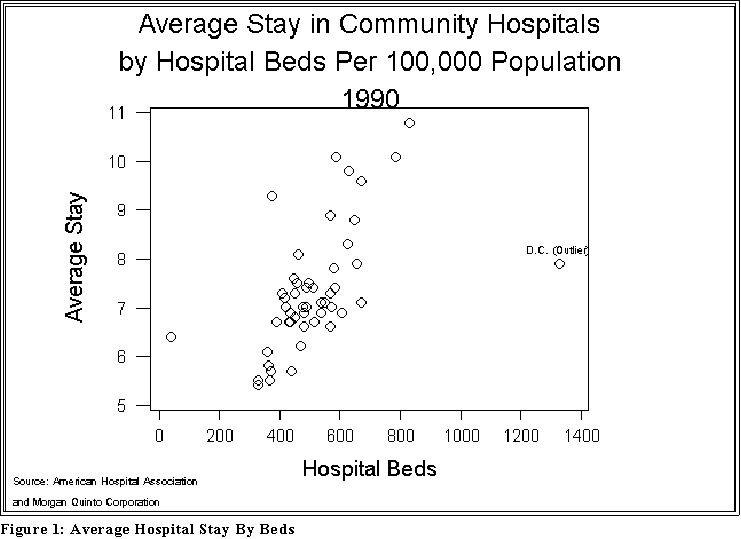1. Here is another example of a scatterplot, this time between the number of beds (X1) and the admission rate (Y2). See Figure 2 below.
1. Interpretation: once again the assertion in the Times article seems to hold water: as the number of beds in a state increases, so too does the number of admissions.
2. Notice also that there is another "extreme" (outlying) point. We will have to adjust the data to take it into account.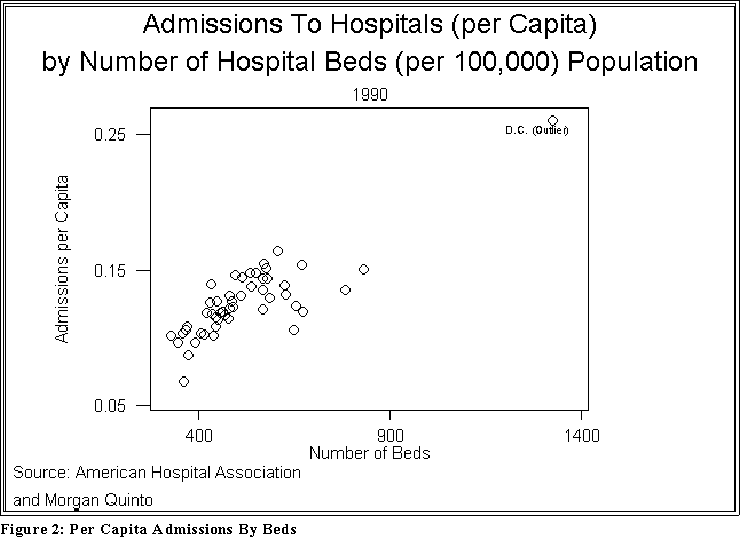1. INTERPRETING PLOTS:
1. The following pages show some different plots and their interpretation:
1. Notice that some of these figures contain equations. Their meaning will be explained later.
2. For now we want to associate a picture with an interpretation.
2. The interpretation of these plots rests on this notion of correlation, a term I am now going to use with a particular meaning.
1. Positive correlation: high values of Y are associated with high values of X and, conversely, low values of Y are associated with low values of X.
2. Negative correlation: high values of Y are associated with low values of X and, conversely, low values of Y are associated with high values of X.

1. For another example of positive non-perfect correlation see the "Smoking and Lung Cancer" Story at
1. http://lib.stat.cmu.edu/DASL/Stories/SmokingandCancer.html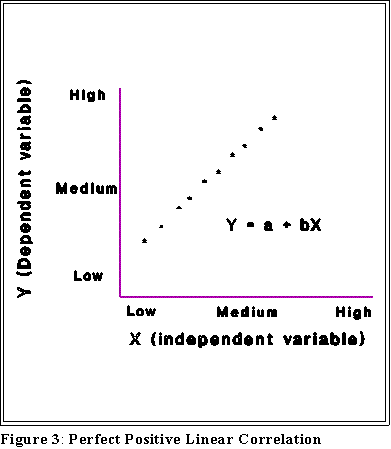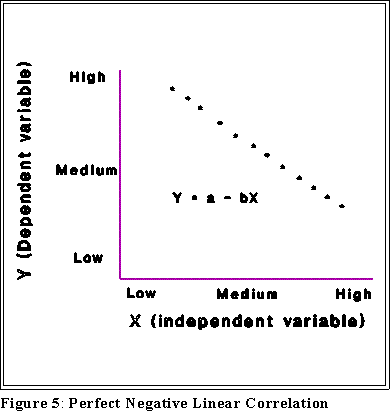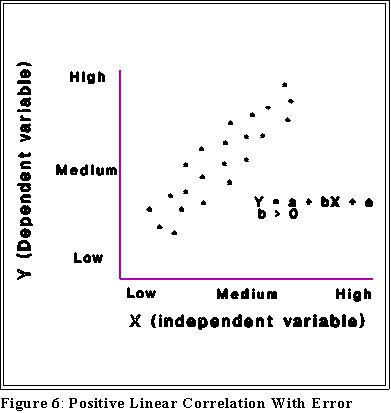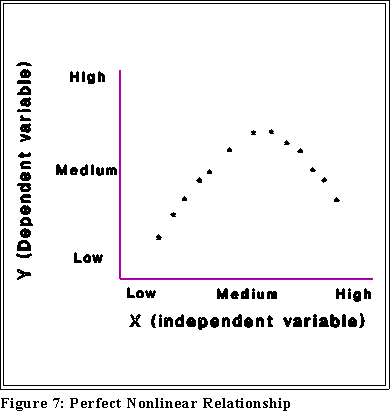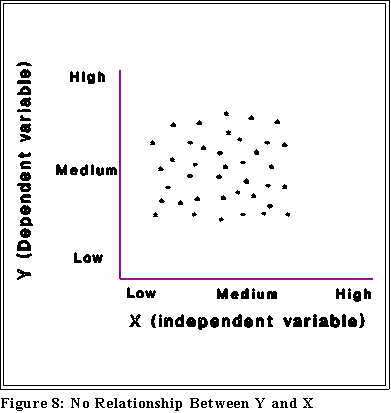1. Note that correlation does not imply causation.

1. MINITAB:
1. Bivariate or scatterplots are produced in MINITAB and SPSS with the Graphics, Plot menu and dialogue box.
1. As with box plots make sure that the independent variable appears in the Y list and the independent variable under X.
2. Use the same options and annotation options as used with box plots.

2. CAUSATION AND CAUSAL ANALYSIS:
1. Some caveats in interpreting X-Y relationships:
1. Causation: Nothing entitles us to assert a causal relationship between X and Y, even if we find a strong relationship.
2. Causation is a matter of inference, not statistics.
3. "Spurious" correlation: X and Y may be related because of a third variable, Z:1. Consider the "Crime Story" at Data and Story Library at Carnegie Mellon
1. The author notes that the relationship between education and crime appears to be positive (i.e., there is a positive correlation), which contradicts commonsense.
1. When two variables are positively or negatively correlated it's important to
1. think carefully about cause and effect
2. consider alternative explanations such as "hidden or lurking variables."
1. Ecological inferences: Much of the data analyzed so far involves geographical units--counties, states, and countries for example--but the statements apply to individuals. Ideally, if one wants to make assertions about individuals, then "individual-level" data should be collected.
1. Be careful, in other words, about saying poverty "causes" illegitimacy (see below) when the data supporting the statement pertain to states, not individuals.
2. Having said this, however, sometimes aggregate data (e.g., states) are the only feasible information and interpretations based on them may be reasonable.
3. The point is simply to be modest and cautious in making claims.
2. Types of relationships
1. Be careful in the use of language and thinking: two variables, X and Y, may be related to some degree.
2. Correlation is a special kind of relationship and a "linear" relationship is a special kind of correlation. To say that two variables are not linearly related does not mean that they are not related in some fashion. (See below)
3. We look for linear relationships because they provide simple and easily understood connections between X and Y. But if the relationship is not linear it may still be associated some other way.
3. Asymmetry:
1. Treating Y as the dependent variable is very much different from treating X as the dependent variable.
2. Do not mix up dependent and independent variables; you get different results in general when Y is dependent.
3. Sometimes you will not know which variable is dependent. It is a statement about your level of knowledge or the level of knowledge in the discipline when the dependent variable is arbitrary.

1. NEXT TIME:
1. Regression, and correlation.